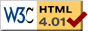### Journée-séminaire de combinatoire

#### (équipe CALIN du LIPN, université Paris-Nord, Villetaneuse)

Le 29 novembre 2011 à 14h00 en B311, Daniel Posner nous parlera de : Efficient solutions for the λ-coloring problem on classes of graphs

Résumé : A λ-coloring of a graph is a function that assigns frequencies (represented by natural numbers) to its vertices in such way that if two vertices are adjacent, then their frequencies differ by at least two, and if two vertices share a common neighbor, then their frequencies must be different. Motivated by mobile wireless networks, the λ-coloring problem aims to use the minimum frequency spectrum without interferences, i.e., respecting the distances restrictions. It is known that the decision version of this problem is NP-complete even for simple classes of graphs such as bipartite graphs, split graphs, and planar graphs. In this seminar we talk about recent results in $lambda$-coloring, including efficient solution when it is restricted to the classes of split permutation graphs and (q, q-4)-graphs, q fixed. Joint work with Marcia R. Cerioli.

 Dernière modification : Monday 24 January 2022Contact pour cette page : Cyril.Banderier at lipn.univ-paris13.fr